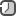### Generators in Python with examplesGenerators allowing you to define a function that can produce individual values one at a time, rather than just a single return value, you have the added flexibility of a function and the performance of an iterator. The most important advantage of this is that it requires very little memory and that it doesn't need to have a predefined size.

Generators are set aside from other functions by their use of the `yield` statement. This is somewhat of an analog to the typical return statement, except that `yield` doesn’t cause the function to stop executing completely. It pushes one value out of the function, which gets consumed by the loop that called the generator, then when that loop starts over, the generator starts back up again. It picks up right where it left off, running until it finds another `yield` statement or the function simply finishes executing.

Here's a simple generator function:

```def simple_generator_function()
yield 1
yield 2
yield 3
```

And here is simple way to use it

```for value in simple_generator_function():
print(value)
1
2
3
```

With the generator in place, you can iterate over the values it produces, simply by treating it like you would any other sequence. Generators are iterable automatically, so a standard `for` loop already knows how to activate it and retrieve its values.

A generator that yields items instead of returning a list

```def seq(n):
num = 0
while num < n:
yield num
num += 1

sum = sum(seq(100))
```

There are multiple ways of creating generators with Python. Generator comprehensions are pretty much identical to list comprehensions but use parentheses instead of brackets, like this for example:

```generator = (x ** 2 for x in range(4))
for x in generator:
print(x)
```

The following are most important advantages of generators

• Memory usage. Items can be processed one at a time, so there is generally no need to keep the entire list in memory.
• The results can depend on outside factors, instead of having a static list.
• Generators are lazy. This means that if you're using only the first five results of a generator, the rest won't even be calculated.
• Generally, it is simpler to write than list generating functions.

The following are most important disadvantages of generators

• The results are available only once. After processing the results of a generator, it cannot be used again.
• The size is unknown until you are done processing, which can be detrimental to certain algorithms.
• Generators are not indexable, which means that `simple_generator_function` will not work.